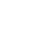Live chatA variance is the diversity involving proposed, budgeted, or average cost and the real cost. This also applies for income. The procedure by which the entire variation involving the average and actual outcome is studied is called variance analysis. The express material procedure variance is the disparity involving the normal capacity of materials which must have been utilized for the amount of units essentially formed and the real measure of materials utilized, estimated at the average cost for each entity of material. Hence, it is the variation between how much material must have been utilized, and how much material was actually utilized, estimated at the average cost (Drury, 2007).

In contrast to absorption costing system, the material usage variances’ account has at least one significant advantage: it counts real material expenditures (whereas the absorption costing system does not). Indeed, it is very important for manufactures as IDP, where row materials’ expenditures (the materials costs, quantity of materials, etc.) take a big part of the total cost. Absorption costing system of IDP takes stock of production overheads for products in total kilograms produced. However, in such a case, it does not take stock of the defective goods, waste or the additional materials’ expenditures. For instance, 1 kilogram of vermilion (needed for production of red paint) costs \$50. To make 100 units of paint the 50 kilograms of vermilion should have been used, or \$2500 (by calculation in absorption costing system).

Although, in the real sense, for every 100 units of paint, 53 kilograms of vermilion were used; an excess of 3 kilograms = \$150. So, from every 100 of units’ usage variance in \$ is \$150, which were not calculated before production. That is why the costs of work-in-progress (WIP) never agree with the book balance. Evidently, stock loss £15,000 for last period in IDP (in the guidelines) - that is total material usage variance, which have been not calculated with absorption costing system.

Furthermore, absorption costing system of IDP makes errors in prices of products. Every month material price variance, fixed overhead volume variance and fixed overhead expenditure variances are calculated. The results of these calculations determine the prices (all these costs are included in the price of every unit). Nevertheless, yet again – the expenditures of real materials’ usage is not calculated and not included in price. For manufactures as IDP the increasing of production may not influence significantly on overhead costs; the row materials’ price is not depended directly from production scale; but with increasing of production the material usage variance would be more appreciable. So, for IDP, the calculation of material usage variances is a more appropriate account system than absorption costing system. For manufactures, where material usage is a very significant expenditure, calculation of material usage variances helps to determine all considerable expenditures (Horngren, 2010).

Limited time Offer

0
0
days
:
0
0
hours
:
0
0
minutes
:
0
0
seconds
Get 19% OFF

The application of a cost systems guarantees that all costs associated with manufacturing are openly allocated or traced to the different products made. The absorption cost system is a costing procedure where the normal costs, whether variable or fixed are charged to the costs of units produced. The marginal cost system is different from the absorption cost system in that period costs are treated as fixed costs.

During production, fixed costs are very important. The most major advantage linked with the absorption cost system is that it identifies the weight of fixed costs by apportioning them to total production of cost units. In addition, when production remains invariable but there is an alteration in sales, the absorption cost system shows less changeability in net profit. However, regardless of these advantages, the absorption cost system is associated with some disadvantages. The absorption cost system has always been regarded as the sole incentive of overproduction. Since fixed costs remain constant despite the produced units, the rate of overheads is lowered following the production of more units (Drury, 2007).

Stay ConnectedMoreover, managers remunerated on total profits planned using absorption cost systems can enhance reported profits by escalating production with the assumption that sales remain constant. However, a variable cost system may decrease the inducement to overproduce by setting aside all fixed manufacturing costs as period costs. Nevertheless, variable cost systems are not normally practical, mostly since their total costs on average go beyond their total benefits.

0

Preparing Orders

0

Active Writers

0

Support Agents

Limited offerget 15% off your 1st order with code first15